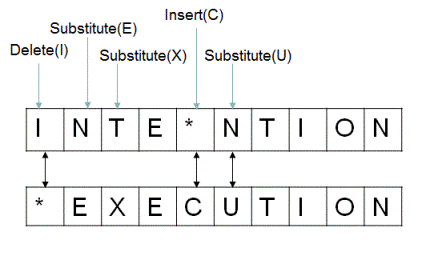## Minimum Edit Distance

Given two strings A and B, find the minimum number of steps required to convert A to B. (each operation is counted as 1 step.) You have the following 3 operations permitted on a word:

• Insert a character
• Delete a character
• Replace a character

Here's a visual representation (source: iDeserve)``# Solve the problem here``
``# Test the solution here``
`` ``
`` ``